Question

# A light ray initially in water enters a transparent substance at an angle of incidence of...

A light ray initially in water enters a transparent substance at an angle of incidence of 47.0°, and the transmitted ray is refracted at an angle of 29.0°. Calculate the speed of light in the transparent substance. Mm/s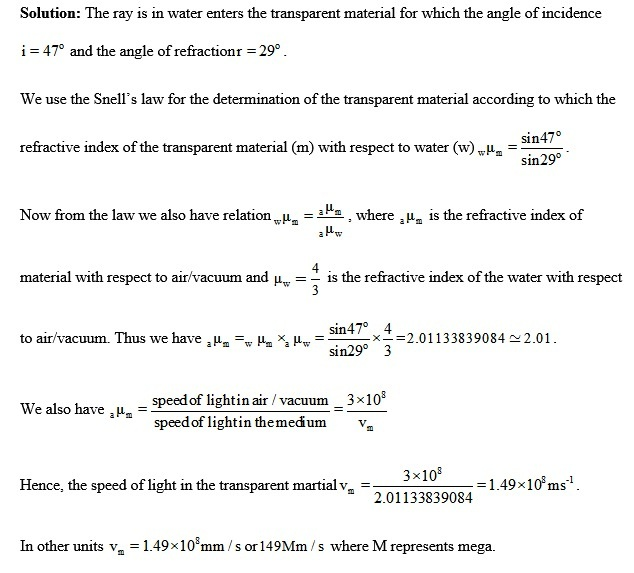#### Earn Coins

Coins can be redeemed for fabulous gifts.

Similar Homework Help Questions
• ### A light ray enters glass from water at an angle of incidence of 30. Calculate the...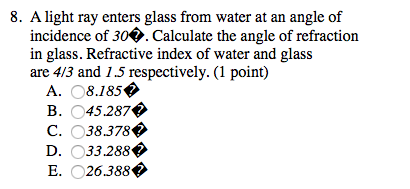A light ray enters glass from water at an angle of incidence of 30. Calculate the angle of refraction in glass. Refractive index of water and glass are 4/3 and 13 respectively. A. 8.185 B. 45.287 C. 38.378 D. 33.288 E. 26.388

• ### A light ray of λ = 490 nm enters at an angle of incidence of 36.6o...

A light ray of λ = 490 nm enters at an angle of incidence of 36.6o from air into a block of plastic. Its angle of refraction is 21.7o. What is the speed of the light inside the plastic?

• ### A ray of light of vacuum wavelength 550 nm traveling in air enters a slab of transparent material

A ray of light of vacuum wavelength 550 nm traveling in air enters a slab of transparent material. The incoming ray makes an angle of 23.0degrees with the normal, and the refracted raymakes an angle of 25.1degrees with the normal. Find the index of refraction of the trans-parent material. (Assume that the index of refraction of air for light of wavelength 550 nm is 1.00.)

• ### A light ray of λ = 570nm enters at an angle of incidence of 36.20 from...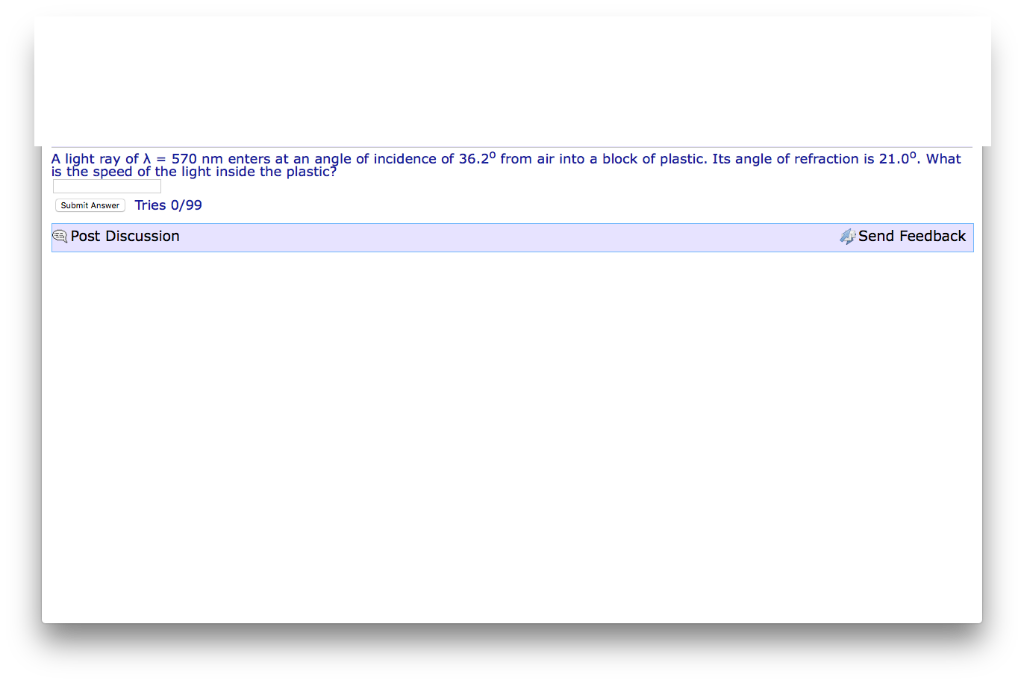A light ray of λ = 570nm enters at an angle of incidence of 36.20 from air into a block of plastic. Its angle of refraction is 21.00, what is the speed of the light inside the plastioc Submit Answer Tries 0/99 Post Discussion Send Feedback

• ### A light ray is incident on the horizontal surface of a transparent tank of water at...

A light ray is incident on the horizontal surface of a transparent tank of water at an angle of 50o . a) Find the refraction angle in the water, b) what is the speed of light in water, c) what is the critical angle for total internal reflection for ray going from water back into the air. d) If an object at the bottom of the tank appears 50 cm deep, what is the true depth?

• ### At an interface between an unknown transparent material and water, a ray of light is incident...

At an interface between an unknown transparent material and water, a ray of light is incident from the water side at an angle of 30.0 degrees to the normal. The refracted ray makes an angle of 25.0 degrees. A) what is the index of refraction for the unknown material? B) what is the critical angle for total internal reflection for the interface described? C) Make a drawing showing the geometry for rays that would undergo total internal reflection at this...

• ### At an interface between an unknown transparent materials and water, a ray of light is incident...

At an interface between an unknown transparent materials and water, a ray of light is incident from the water side at an angle of 45 degree to the normal. The refracted ray makes an angle of 36.8 degree with the normal. A) What is the index of refraction for the unknown materials? B) what is the critical angle for total internal reflection for the interface described? C)  make a drawing showing the geometry for rays that would undergo total internal reflection...

• ### A ray of light enters one end of an optical fiber with an angle of incidence...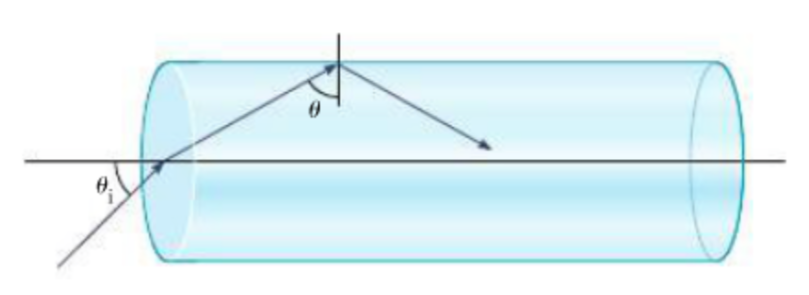A ray of light enters one end of an optical fiber with an angle of incidence θi = 50.0◦. The index of refraction of the fiber is 1.62. a. Find the angle θ the ray makes with the normal when it reaches the curved surface of the fiber. b. Show that the ray inside the fiber is undergoing total internal reflection. Why would you want this inside an optical fiber? θ

• ### A light ray passes from water into ice. If the transmitted ray makes an angle of...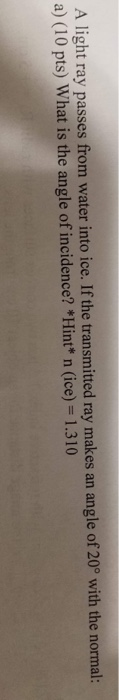A light ray passes from water into ice. If the transmitted ray makes an angle of 20° with the normal a) (10 pts) What is the angle of incidence? *Hint* n (ice) 1.310

• ### A ray of light propagates in air, entering a rectangular quartz prism with index of refraction...

A ray of light propagates in air, entering a rectangular quartz prism with index of refraction 1.63 at an incidence angle of 60⁰. After it passes through the quartz, it enters a fluid with index of refraction 1.34. (a) What is the refracted angle when the light enters the quartz? ⁰ (b) What is refracted angle of the light when it enters the fluid? ⁰ (c) How much does the light speed up when it enters the fluid? m/s